## Approach to Weekly 183

Here is an approach to Weekly 183.

 1. This puzzle is quite hard due to the number of large cages in it. Start by looking at what cannot be in the large cages. For example, the 42-cage in the upper right cannot contain 1 or 2. And the 39-cage in the upper-right cannot contain a 1, 2, or 3. (since it is a 6-cage summing to 39). Thus, the red cells must contain 1 and 2.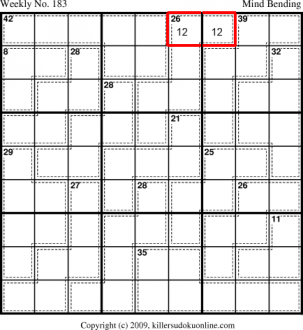2. And, since the 42-cage cannot contain 1 or 2 and the 26-cage with the red cells already has 1 and 2 in it, the blue cell must be 1 or 2.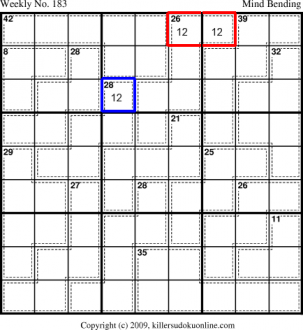3. The rule of 45 indicates the blue cells must be 27 or 28. If they sum to 28, then they are: - 4789 or - 5689 and, then red cells contain 1 and 3 and something else. If they sum to 27, then they are: - 4689 or - 5679 and, then the red cells contain 2 and 3 and something else.4. The 26-cage has 4 unknown cells. They sum to 23 with a 1 or 2: - 3479 - 3569 - 3578 - 4568 If the 8-cage (in red) is 134, then the blue cell must contain a 3 or 4. If the 8-cage is 125, then the the blue cell must contain a 5 or 3479.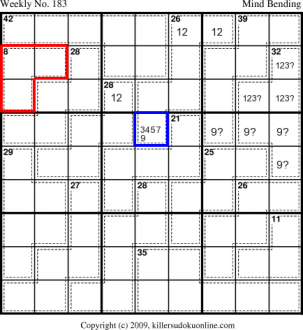5. The blue cells must sum to 14 (rule of 45 combined with knowing the red cells will both be 1 or both be 2.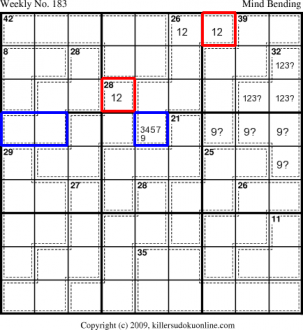6. The blue cell at C5R6 cannot contain a 9. If it did then, the blue cell at C9R5 would have to a 9. and the only possible total for the red cells of the 28-cage would be 26 (the only way not to include a 9). But in that case there is no cell in row six which could contain a 3.7. By the rule of 45, the blue cells must be 23 more than the reed cell. Thus, the red cell must be a 1.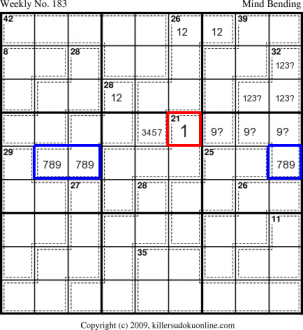8. From this we can now set the red cells. And, we can remove 1 from the blue cells.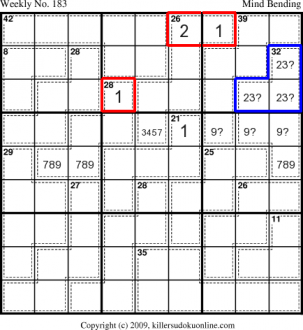9. Since the red cells must sum to 27 (and they cannot contain a 3) we know the top three cells must contain a 6. Thus, the blue cells, which also sum to 27, cannot contain a 6. They must then be 3789.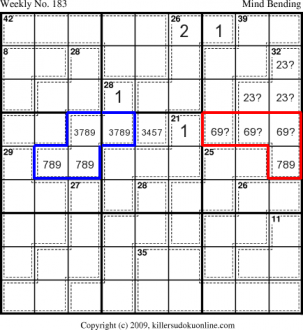10. Due to the 3 in the 38-cage, we know that the red cell cannot have a 3.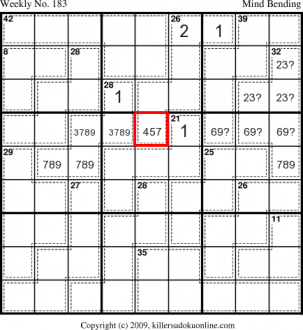11. The red cells sum to 14. (See step 5). It cannot contain 1, 3, or 6. This leaves: 257 or 248. However, 248 would be illegal, since the blue cells must contain an 8. Thus, the red cells must be 2+5+7. Furthermore, the 7 must be in column 5, due to the need for a 7 in the blue cells.12. Some clean up.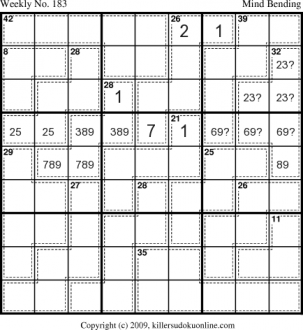13. Since the 42-cage cannot contain a 2 and the 28-cage (both in the upper-left nonet) already has a 2, we know the 8-cage must be 125.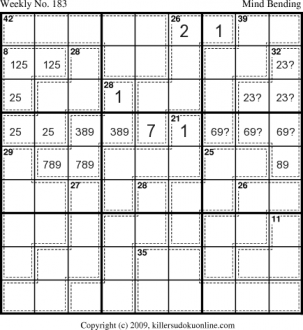14. The red cells sum to 21 and they cannot contain 1, 2, or 5. Thus, they must be either: 489 or 678. In other words, they must contain an 8. This means the blue region must contain an 8. And the 26-cage to the right of the 42-cage cannot contain an 8. Since it already has a 1, 2, and 7 the only legal sum is 123479.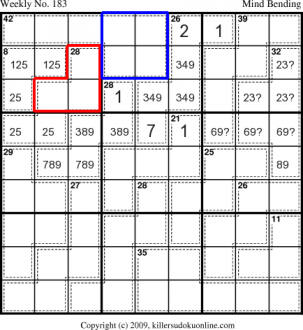15. We can now eliminate lots of possibilities in the top left and top middle nonet.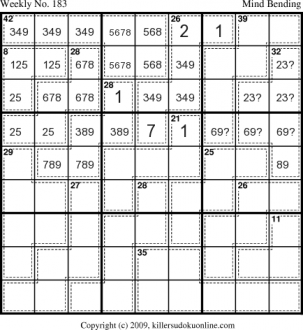16. The red cells force the blue cell to be a 1.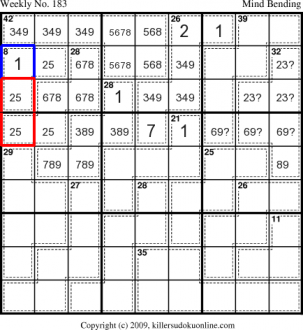17. The use of 2357 in the sixth row limits the possible sum of the red region to 4689.18. The rule of 45 makes the red cell equal to the blue cell.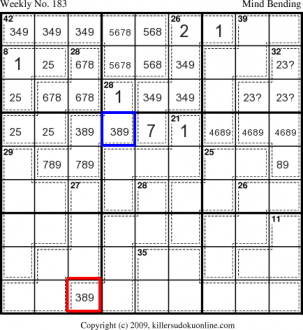19. The blue cells force the cells in the third column of the lower left nonet to contain 25. Since the bottom cell doesn't contain either the red cells must be 25.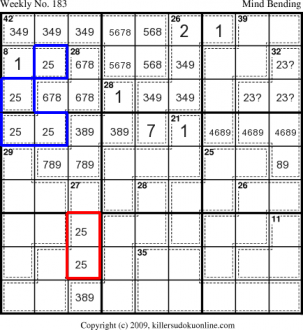20. The rule of neccesity forces the red cell to be a 1.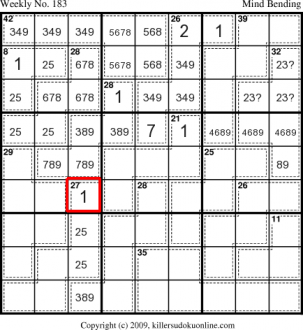21. The only place left for a 1 in the bottom left nonet is the red cell.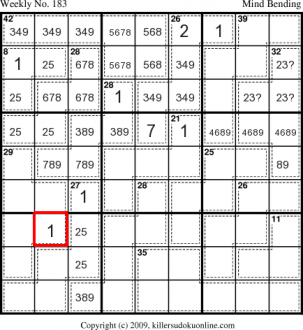22. The red cells in the middle left nonet must contain a 4 and 6. Thus, the blue cells must also contain a 4 and 6. This forces the blue cells, which must sum to 19 to be 469.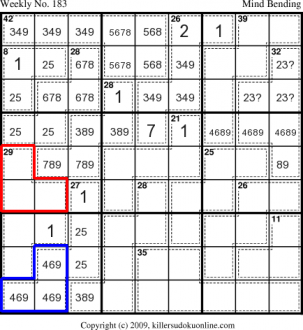23. Some clean up. The red cell cannot be a 9. We know then (from step 18) that the blue cell cannot be a 9.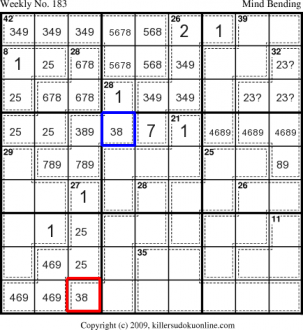24. The rule of necessity makes the red cell a 4. Likewise, the blue cells must contain a 6.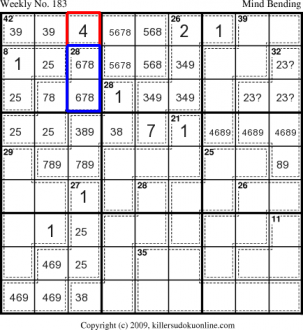25. The only place for a 1 in the middle right nonet is the red cell.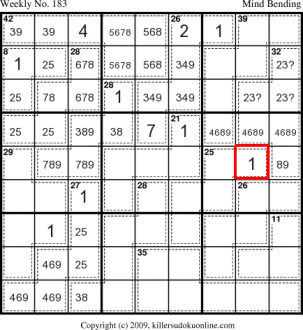26. The bottom midle nonet must have a 1 in the red cells. Which means the bottom right nonet must have a 1 in the blue cell.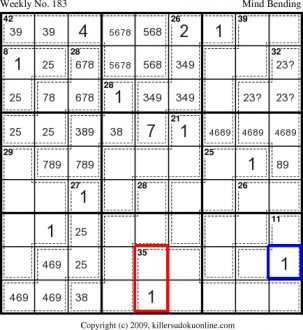27. The red cells in the middle nonet must sum to 17. The only valid sums are: - 359 - 368 - 458 Thus the blue cell must be 8 or 9. And the a 2 must be in row 5.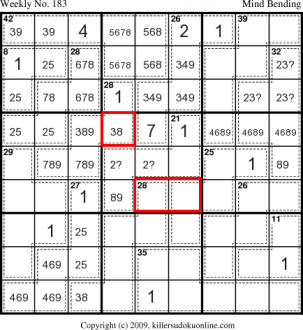28. The red cells in the middle nonet must sum to 17. The only valid sums are: - 359 - 368 - 458 Thus the blue cell must be 8 or 9. And the a 2 must be in row 5.29. Using the information we have we can fill in the possibilities for the blue cells.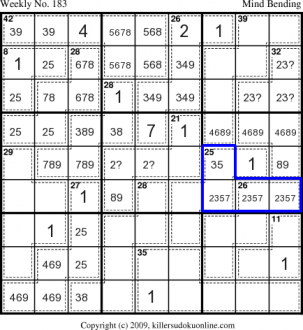30. The 28-cage in red cannot contain 1 or 7. Thus, it must be 234568. From this we can see that the red cell cannot be an 8.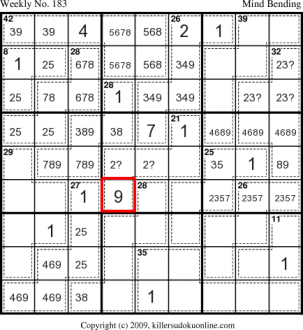31. The red cells eliminate the possibility of a 9 in the blue cell.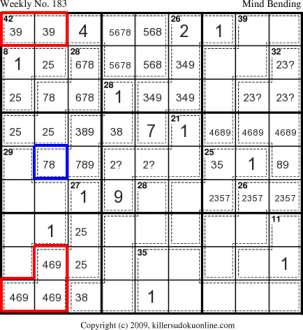32. The red cells sum to 24. The possible sums are: - 2589 - 2679 Not possible - 3489 Not possible - 3579 Not possible - 3678 - 4569 Not possible - 4578 The remaining possible sums are: - 2589 - 3678 - 4578 Looking at these we see that the only place for a 7 in the bottom nonet is in the blue cells.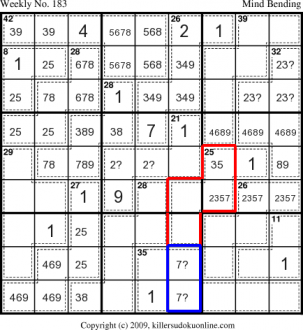33. The possible sums also determine that the red cell cannot contain either 3 or 5.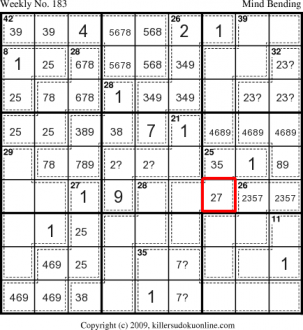34. To make the 29-cage on the left the red cells must be 46 and either 3 or 8. The blue cells must be 7 and either 3 or 8.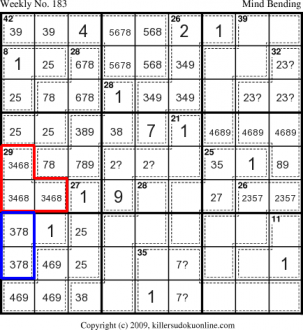35. The red cells must sum to 11 (45-(24+10)), so C1R5 must be 4 or 6.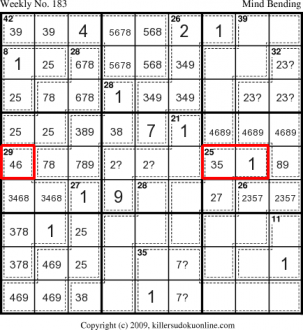36. The red cells eliminate an 8 from the blue cell.37. The rule of 45 indicates that the red cells have to be 9 more than the blue cell. Using this we can see that there is no possible way for a 9 to be in C1R9.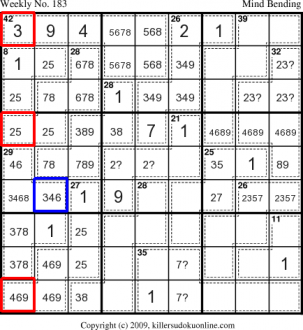38. Some basic elimination can now be applied.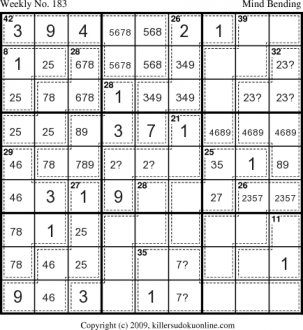39. The red cells must sum to 14.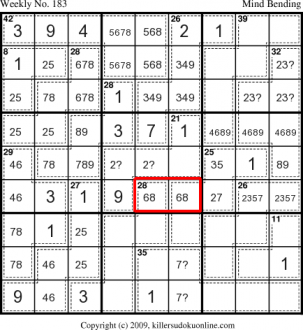40. Basic elimination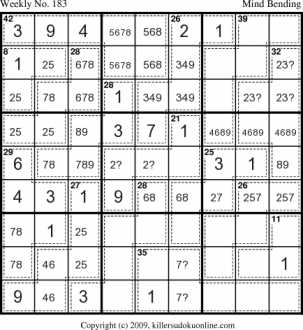41. Rule of necessity.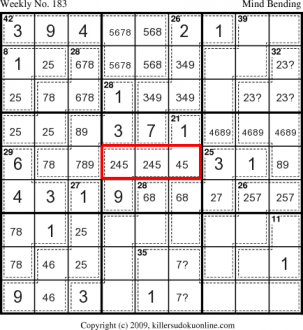42. The blue cells sum to 21, so the 2 is illegal. And, then there is only one way to finish this cage.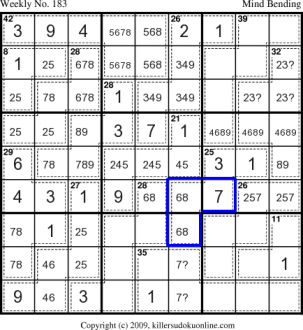43. Basic Eliminationbbb77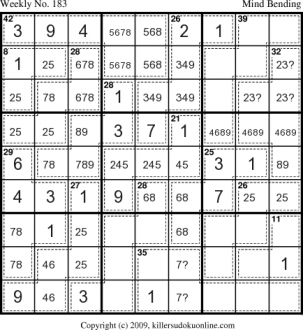44. The 28-cage in red cannot contain a 9, so the blue region must contain a 9. Since the 11-cage to the right cannot contain a 9, we know that a 9 must be in one of the cells in the red cells of the bottom right nonet. If we look at the possible sums for 26-cage on the right we see it must include 469 in the red cells.45. This means the 11-cage must be 137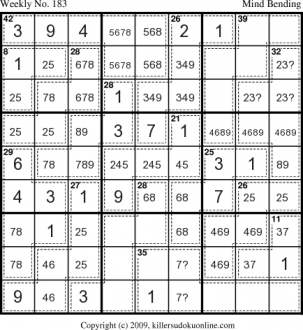46. This leaves 258 for the red region and 379 for the blue region.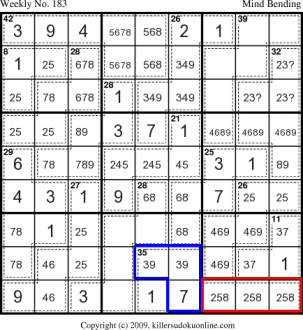47. Basic Elimination.bbb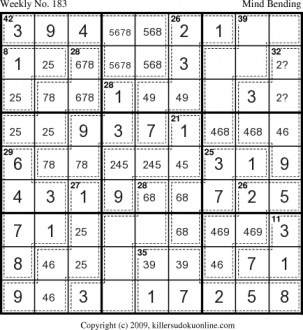48. Rule of necessity and basic elimination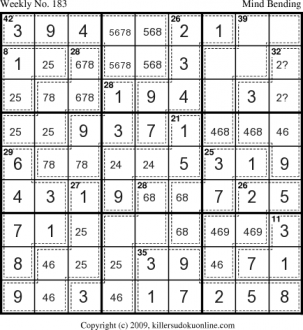49. If you have followed along to this point, the rest of the puzzle should fall into place.